Next: The Born-Oppenheimer Approximation Up: Molecular Quantum Mechanics Previous: Molecular Quantum Mechanics

## The Molecular Hamiltonian

We have noted before that the kinetic energy for a system of particles is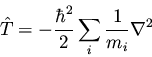(132)

The potential energy for a system of charged particles is
(133)

For a molecule, it is reasonable to split the kinetic energy into two summations--one over electrons, and one over nuclei. Similarly, we can split the potential energy into terms representing interactions between nuclei, between electrons, or between electrons and nuclei. Using i and j to index electrons, and A and B to index nuclei, we have (in atomic units)
(134)

where
,
, and
. This is known as the exact'' nonrelativistic Hamiltonian in field-free space. However, it is important to remember that this Hamiltonian neglects at least two effects. Firstly, although the speed of an electron in a hydrogen atom is less than 1% of the speed of light, relativistic mass corrections can become appreciable for the inner electrons of heavier atoms. Secondly, we have neglected the spin-orbit effects. From the point of view of an electron, it is being orbited by a nucleus which produces a magnetic field (proportional to L); this field interacts with the electron's magnetic moment (proportional to S), giving rise to a spin-orbit interaction (proportional to
for a diatomic.) Although spin-orbit effects can be important, they are generally neglected in quantum chemical calculations.Next: The Born-Oppenheimer Approximation Up: Molecular Quantum Mechanics Previous: Molecular Quantum Mechanics